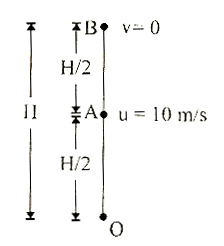# A ball is thrown vertically upward. It has a speed of 10 m/sec when it has reached one half of its maximum height. How high does the ball rise? Take g = 10 m/s2 (a)    10 m         (b)    5 m (c)    15m (d)    20m

8 years ago

(a)
For part AB
From 3rd equation of motion v2 = u2 - 2gH

0=u^2-2g (H/2)=u^2-gH
H=   u^2/g=  ?10?^2/10   =10 mone year ago

For part AB
From 3rd equation of motion v2 = u2 - 2gH

0=u^2-2g (H/2)=u^2-gH
H=   u^2/g=  ?10?^2/10   =10 m